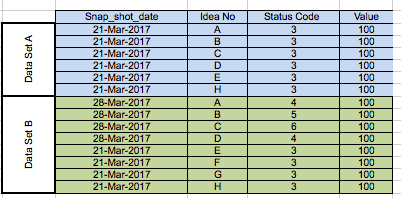New to QlikView

Discussion board where members can get started with QlikView.

HighlightedNot applicable

Expression Inside a Expression

Hello

Problem : - I want to compare data from 2 dates, I have 2 date selection filters in my dashboard -

a) From_Date and

b) Current_Report_Date,

( both actually picks from Snap_shot_date field - see below sample data)

I always compare the Current_Report_Date to From_Date.

Sample Data

Here From_Date = 21 Mar 2017 and Current_Report_Date = 28 Mar 2017 ( so I am essentially comparing 28th to 21st Mar)Manually I do the following to get my answer

Step 1 : - Find Ideas that appeared on both dates then I get A,B,C,D,E,H

Step 2:    Filter results of Step 1 to only those ideas that has  progressed in the status code or has gone greater than 3 on the latter date(Current_Report_Date) , now we get A,B,C,D

Step 3:  Sum Value of ideas that you get in step 2 to get =\$400

Set Expression ( doesn't seem to work, I am unsure whether this is right to use the P() function ).

Sum(

{< Status_Code = {4,5,6}, SNAP_SHOT_DATE = { Current_Report_Date} ,

Idea_No = P( only({<SNAP_SHOT_DATE = {'\$(=[From_Date])'} ,Status_Code = {3}>})  Idea_No) >}

) Savings

2 RepliesMVP

Re: Expression Inside a Expression

Is Current_Report_Date a field that you selected (and From_Date is a variable)? Then this should work

Sum({

<

SNAP_SHOT_DATE = {"\$(=Date(Max(Current_Report_Date)))"},

Status_Code = {4,5,6}

>

*

<

SNAP_SHOT_DATE = {"\$(=From_Date)"},

Status_Code = {3}

>

) Savings

If Current_Report_Date is a variable, then the first SNAP_SHOT_DATE line could be

SNAP_SHOT_DATE = {"\$(=Current_Report_Date)"},

If these don't work, I suggest you post a small sample qvw file for more detailed help.

Logic will get you from a to b. Imagination will take you everywhere. - A Einstein
Esteemed Contributor II

Re: Expression Inside a Expression

see attached

=Sum (

{<Idea = p({<DataSet ={'A'} >} Idea)>*<Idea = p({<DataSet ={'B'},StatusCode={">3"} >} Idea)

>}

Value)